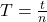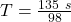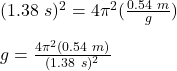## After landing on an unfamiliar planet, a space explorer constructs a simple pendulum of length 54.0 cm. The explorer finds that the pendulum

Question

After landing on an unfamiliar planet, a space explorer constructs a simple pendulum of length 54.0 cm. The explorer finds that the pendulum completes 98.0 full swing cycles in a time of 135s.

Required:
What is the value of g on this planet?

in progress 0
4 weeks 2021-08-17T12:55:58+00:00 1 Answers 2 views 0

g = 11.2 m/s²

Explanation:

First, we will calculate the time period of the pendulum:where,

T = Time period = ?

t = time taken = 135 s

n = no. of swings in given time = 98

Therefore,T = 1.38 s

Now, we utilize the second formula for the time period of the simple pendulum, given as follows:where,

l = length of pendulum = 54 cm = 0.54 m

g = acceleration due to gravity on the planet = ?

Therefore,g = 11.2 m/s²